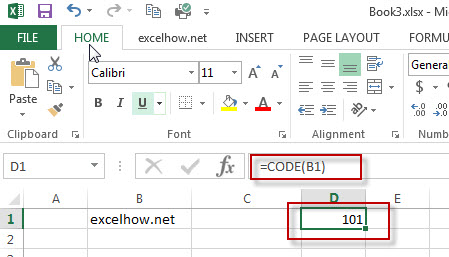# Excel Code Function

This post will guide you how to use Excel CODE function with syntax and examples in Microsoft excel.

### Description

The Excel CODE function returns the numeric ASCII value for the first character of a text string.

The CODE function is a build-in function in Microsoft Excel and it is categorized as a Text Function.

The CODE function is available in Excel 2016, Excel 2013, Excel 2010, Excel 2007, Excel 2003, Excel XP, Excel 2000, Excel 2011 for Mac.

### Syntax

The syntax of the CODE function is as below:

= CODE  (text)

Where the CODE function argument is:
Text – this is a required argument. The text strings that you want to get the numeric value of the first character

### Example

The below examples will show you how to use Excel CODE Text function to get the numeric value of the first character in a cell.

#1 To get the numeric ASCII value of the first character in B1 cell, just using CODE(B1) formulas.